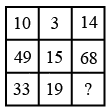Question 8

# Study the given pattern carefully and select the number that can replace the question mark (?) in it.Solution

The logic here is

$$\left(10-3\right)\times2=14$$

$$\left(49-15\right)\times2=68$$

Along the rows, twice the difference of first number and second number is equal to third number.

Similarly from the third row, the required number = $$\left(33-19\right)\times2=28$$

Hence, the correct answer is Option B